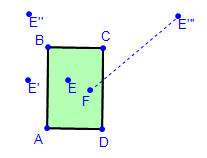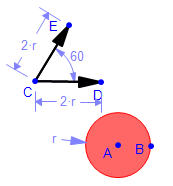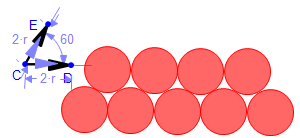Math Illustrations Tips
 July 2011
 This month's tips are all about transformations.  We'll find out how to reflect in a point, how to use reflections to create the ideal path for a billiard ball, and, staying on the pool table, how to use translations to quickly draw 15 pool palls racked up.We're on the web at www.mathillustrations.com.Billiard Reflections [Video] Given two balls on a billiard table, what path will take one ball to the other while first bouncing off 3 cushions?Given the two points E and F, and the table ABCD, reflect E in AB, then reflect the image E' in a BC, then reflect its image E'' in CD. Draw a line segment connecting F with E'''. (make it dotted to distinguish it as a construction line).Now reflect the line FE''' in CD, then reflect F'E'' in BC, then reflect F'''E in AB.Add new line segments which join E to F via the intersections between the construction lines and the sides of the table.Now you can hide the construction lines to leave only the ball's path.For more reflection based models, look here , here and here .
 Pool Translations [Video]We'll use translations to create a picture of the triangular arrangement of pool balls at the start of a game. Draw a circle representing a ball.  Specify its radius to be r. We are going to create translated copies of this ball.  We want to translate either by distance 2r horizontally or at an angle of 60 degrees.  So we create two vectors, one horizontal, and one at 60 degrees, and make the length of each 2*r.Now we translate the original ball using the horizontal vector, and repeat translating the new ball till we have 5 balls arranged in a horizontal line.Now we select the first 4 balls and translate using the diagonal vector.We repeat selecting 3 then 2 then 1 ball from the next 3 lines, eventually yielding the triangle of balls.Point Reflections Math Illustrations offers a full set of Euclidean transformations:  translations, rotations, reflections, and dilations.But reflections are reflections in a line.  What if you want to reflect an object in a point?There are two equivalent transformations which you can use to reflect in a point:Rotate by 180 degrees about the pointDilate by a factor of -1 with a center at the point.